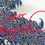# Gravity on Earth

On Earth, the equation for gravitational force functions the same as the rest of the universe, but we know exactly what m2 is - it's the mass of the Earth. This quantity, generally written as mE when put into the formula, is 5.97 x 1024 kg. If we're talking about gravity on the surface of the Earth, then we use the radius of the Earth as the value r, which is generally referenced as RE (and has a value of 6.38 x 106 m).

Weight vs. Mass

It is easy to confuse mass and weight, because most of the world uses the metric convention of measuring mass instead of weight. We Americans, therefore, get confused over the difference between kilograms and pounds, even though these measure different quantities. Pounds are a measure of the gravitational force on us and it changes when we, for example, travel to the moon, where we use the moon's mass instead of the Earth's to calculate weight. Mass only changes if we go on a diet and/or exercise. A change in mass will result in a change in weight, however, and you can determine the mass of an object by measuring it's weight and applying the aforementioned gravity formula to obtain its mass. Acceleration Due to Gravity

Newton's Laws of Motion tell us that force is proportional to the product of mass and acceleration. Therefore, we can divide both sides of the equation by the individual object's mass to obtain an equation for the acceleration caused by gravity, g.

Since we know all of the values on the right side of this equation, we obtain a constant gravitational acceleration on the surface of the Earth, which comes out to 9.80 m/s2 (note that here m stands for the unit meters, not a variable for mass).

These equations assume that you're at or relatively near the Earth's surface. Obviously, the further away you get, the less accurate RE is as an approximation. The required level of precision for your work will determine if you can use this value for g or whether you will need to calculate it directly with a more specific value of r.

Shortcut to Weight & Force

In most physics problems in the classroom, we refer to the gravitational force as simply the product of mass and gravitational acceleration, Fg = mg. Similarly, since weight is a measurement of the force of gravity, w = mg. Again, this does not work in cases where approximating the location as the Earth's surface doesn't obtain the proper level of precision. But 99% of the time, if your problem takes place on the Earth, you will be using this formula to obtain the force of gravity (or weight). In these cases, you'll have to take account exact position to get your precise value, using the more comprehensive theory of universal gravitation directly.

Gravitational Potential Energy

Many problems involving gravity can be solved by applying the concept of gravitational potential energy. Since potential energy is a relative quantity, and problems generally require knowing the change in the potential energy rather than the absolute potential energy, the equation for gravitational potential energy U near the Earth's surface is straightforward. It is defined in term of the object's mass m, the acceleration of gravity g, and the distance y above the coordinate origin (generally the ground) in the form: U = mgy This equation can be applied in conjunction with kinetic energy to determine how one quantity changes if the total energy remains the same.Note by Madison Jones
5 years, 9 months ago

This discussion board is a place to discuss our Daily Challenges and the math and science related to those challenges. Explanations are more than just a solution — they should explain the steps and thinking strategies that you used to obtain the solution. Comments should further the discussion of math and science.

When posting on Brilliant:

• Use the emojis to react to an explanation, whether you're congratulating a job well done , or just really confused .
• Ask specific questions about the challenge or the steps in somebody's explanation. Well-posed questions can add a lot to the discussion, but posting "I don't understand!" doesn't help anyone.
• Try to contribute something new to the discussion, whether it is an extension, generalization or other idea related to the challenge.
• Stay on topic — we're all here to learn more about math and science, not to hear about your favorite get-rich-quick scheme or current world events.

MarkdownAppears as
*italics* or _italics_ italics
**bold** or __bold__ bold
- bulleted- list
• bulleted
• list
1. numbered2. list
1. numbered
2. list
Note: you must add a full line of space before and after lists for them to show up correctly
paragraph 1paragraph 2

paragraph 1

paragraph 2

[example link](https://brilliant.org)example link
> This is a quote
This is a quote
    # I indented these lines
# 4 spaces, and now they show
# up as a code block.

print "hello world"
# I indented these lines
# 4 spaces, and now they show
# up as a code block.

print "hello world"
MathAppears as
Remember to wrap math in $$ ... $$ or $ ... $ to ensure proper formatting.
2 \times 3 $2 \times 3$
2^{34} $2^{34}$
a_{i-1} $a_{i-1}$
\frac{2}{3} $\frac{2}{3}$
\sqrt{2} $\sqrt{2}$
\sum_{i=1}^3 $\sum_{i=1}^3$
\sin \theta $\sin \theta$
\boxed{123} $\boxed{123}$

## Comments

There are no comments in this discussion.

×

Problem Loading...

Note Loading...

Set Loading...